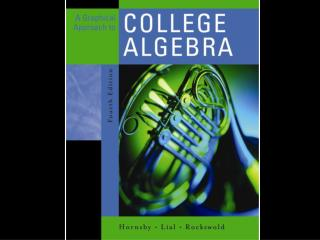# Chapter 8: Further Topics in Algebra - PowerPoint PPT PresentationDownload PresentationChapter 8: Further Topics in Algebra

Chapter 8: Further Topics in Algebra
Download Presentation## Chapter 8: Further Topics in Algebra

- - - - - - - - - - - - - - - - - - - - - - - - - - - E N D - - - - - - - - - - - - - - - - - - - - - - - - - - -
##### Presentation Transcript

1. Chapter 8: Further Topics in Algebra 8.1 Sequences and Series 8.2 Arithmetic Sequences and Series 8.3 Geometric Sequences and Series 8.4 The Binomial Theorem 8.5 Mathematical Induction 8.6 Counting Theory 8.7 Probability

2. 8.7 Probability Basic Concepts • An experiment has one or more outcomes. The outcome of rolling a die is a number from 1 to 6. • The sample space is the set of all possible outcomes for an experiment. The sample space for a dice roll is {1, 2, 3, 4, 5, 6}. • Any subset of the sample space is called an event. The event of rolling an even number with one roll of a die is {2, 4, 6}.

3. 8.7 Probability Probability of an Event E In a sample space with equally likely outcomes, the probability of an event E, written P(E), is the ratio of the number of outcomes in sample space S that belong to E, n(E), to the total number of outcomes in sample space S, n(S). That is,

4. 8.7 Finding Probabilities of Events Example A single die is rolled. Give the probability of each event. (a) E3 : the number showing is even (b) E4 : the number showing is greater than 4 (c) E5 : the number showing is less than 7 (d) E6 : the number showing is 7

5. 8.7 Finding Probabilities of Events Solution The sample space S is {1, 2, 3, 4, 5, 6} so n(S) = 6. (a) E3 = {2, 4, 6} so (b) E4= {5, 6} so

6. 8.7 Finding Probabilities of Events Solution (c) E5 = {1, 2, 3, 4, 5, 6} so (b) E6 = Ø so

7. 8.7 Probability • For an event E, P(E) is between 0 and 1 inclusive. • An event that is certain to occur always has probability 1. • The probability of an impossible event is always 0.

8. 8.7 Complements and Venn Diagrams • The set of all outcomes in a sample space that do not belong to event E is called the complement of E, written E´. If S = {1, 2, 3, 4, 5, 6} and E = {2, 4, 6} then E´ = {1, 3, 5}.

9. 8.7 Complements and Venn Diagrams • Probability concepts can be illustrated with Venn diagrams. The rectangle represents the sample space in an experiment. The area inside the circle represents event E; and the area inside the rectangle but outside the circle, represents event E´.

10. 8.7 Using the Complement Example A card is drawn from a well-shuffled deck, find the probability of event E, the card is an ace, and event E´. Solution There are 4 aces in the deck of 52 cards and 48 cards that are not aces. Therefore

11. 8.7 Odds The odds in favor of an event E are expressed as the ratio of P(E) to P(E´) or as the fraction

12. 8.7 Finding Odds in Favor of an Event Example A shirt is selected at random from a dark closet containing 6 blue shirts and 4 shirts that are not blue. Find the odds in favor of a blue shirt being selected. SolutionE is the event “blue shirt is selected”.

13. 8.7 Finding Odds in Favor of an Event Solution The odds in favor of a blue shirt are or 3 to 2.

14. 8.7 Probability Probability of the Union of Two Events For any events E and F,

15. 8.7 Finding Probabilities of Unions Example One card is drawn from a well-shuffled deck of 52 cards. What is the probability of each event? (a) The card is an ace or a spade. (b) The card is a 3 or a king.

16. 8.7 Finding Probabilities of Unions Solution (a) P(ace or space) = P(ace) + P(spade) – P(ace and spade) (b) P(3 or K) = P(3) + P(K) – P(3 and K)

17. 8.7 Probability Properties of Probability 1. 2. P(a certain event) = 1; 3. P(an impossible event) = 0; 4. 5.

18. 8.7 Binomial Probability An experiment that consists of • repeated independent trials, • only two outcomes, success and failure, in each trial, is called a binomial experiment.

19. 8.7 Binomial Probability Let the probability of success in one trial be p. Then the probability of failure is 1 – p. The probability of r successes in n trials is given by

20. 8.7 Finding Binomial Probabilities Example An experiment consists of rolling a die 10 times. Find the probability that exactly 4 tosses result in a 3. Solution Here , n = 10 and r = 4. The required probability is